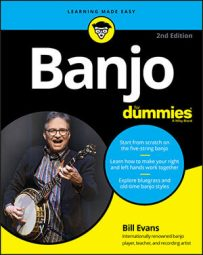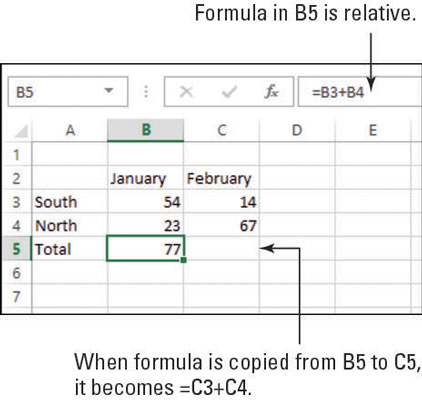##### Banjo For DummiesIn Excel 2013 you can move and copy text and numbers between cells, but when it comes to copying formulas, beware of a few gotchas. The following sections explain relative and absolute referencing in formulas and how you can use them to get the results you want when you copy.

## Copy formulas with relative referencing

When you move or copy a formula, Excel automatically changes the cell references to work with the new location. That’s because, by default, cell references in formulas are relative references.

For example, in this figure, suppose you wanted to copy the formula from B5 into C5. The new formula in C5 should refer to values in column C, not to column B; otherwise the formula wouldn’t make much sense. So, when B5’s formula is copied to C5, it becomes =C3+C4 there.A relative reference is a cell reference that changes if copied to another cell.

## Copy formulas with absolute referencing

You might not always want the cell references in a formula to change when you move or copy it. In other words, you want an absolute reference to that cell. To make a reference absolute, you add dollar signs before the column letter and before the row number. So, for example, an absolute reference to cell C1 would be =\$C\$1.

An absolute reference is a cell reference that doesn’t change when copied to another cell. You can mix relative and absolute references in the same formula. When you do, the result is a mixed reference.

If you want to lock down only one dimension of the cell reference, you can place a dollar sign before only the column or only the row. For example, =\$C1 would make only the column letter fixed, and =C\$1 would make only the row number fixed.